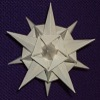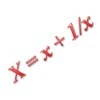# Resources tagged with: Polynomial functions and their roots

Filter by: Content type:
Age range:
Challenge level:

### There are 23 results

Broad Topics > Coordinates, Functions and Graphs > Polynomial functions and their roots### Polynomial Interpolation

##### Age 16 to 18Challenge Level

Can you fit polynomials through these points?### Telescoping Functions

##### Age 16 to 18

Take a complicated fraction with the product of five quartics top and bottom and reduce this to a whole number. This is a numerical example involving some clever algebra.### Mechanical Integration

##### Age 16 to 18Challenge Level

To find the integral of a polynomial, evaluate it at some special points and add multiples of these values.### Real(ly) Numbers

##### Age 16 to 18Challenge Level

If x, y and z are real numbers such that: x + y + z = 5 and xy + yz + zx = 3. What is the largest value that any of the numbers can have?### More Polynomial Equations

##### Age 16 to 18Challenge Level

Find relationships between the polynomials a, b and c which are polynomials in n giving the sums of the first n natural numbers, squares and cubes respectively.### Two Cubes

##### Age 14 to 16Challenge Level

Two cubes, each with integral side lengths, have a combined volume equal to the total of the lengths of their edges. How big are the cubes? [If you find a result by 'trial and error' you'll need to. . . .### Interpolating Polynomials

##### Age 16 to 18Challenge Level

Given a set of points (x,y) with distinct x values, find a polynomial that goes through all of them, then prove some results about the existence and uniqueness of these polynomials.### Symmetrically So

##### Age 16 to 18Challenge Level

Exploit the symmetry and turn this quartic into a quadratic.### Agile Algebra

##### Age 16 to 18Challenge Level

Observe symmetries and engage the power of substitution to solve complicated equations.### An Introduction to Galois Theory

##### Age 16 to 18

This article only skims the surface of Galois theory and should probably be accessible to a 17 or 18 year old school student with a strong interest in mathematics.### Common Divisor

##### Age 14 to 16Challenge Level

Find the largest integer which divides every member of the following sequence: 1^5-1, 2^5-2, 3^5-3, ... n^5-n.### The Why and How of Substitution

##### Age 16 to 18

Step back and reflect! This article reviews techniques such as substitution and change of coordinates which enable us to exploit underlying structures to crack problems.##### Age 16 to 18Challenge Level

In y = ax +b when are a, -b/a, b in arithmetic progression. The polynomial y = ax^2 + bx + c has roots r1 and r2. Can a, r1, b, r2 and c be in arithmetic progression?### Poly Fibs

##### Age 16 to 18Challenge Level

A sequence of polynomials starts 0, 1 and each poly is given by combining the two polys in the sequence just before it. Investigate and prove results about the roots of the polys.### Polynomial Relations

##### Age 16 to 18Challenge Level

Given any two polynomials in a single variable it is always possible to eliminate the variable and obtain a formula showing the relationship between the two polynomials. Try this one.### Cubic Spin

##### Age 16 to 18Challenge Level

Prove that the graph of f(x) = x^3 - 6x^2 +9x +1 has rotational symmetry. Do graphs of all cubics have rotational symmetry?### Janine's Conjecture

##### Age 14 to 16Challenge Level

Janine noticed, while studying some cube numbers, that if you take three consecutive whole numbers and multiply them together and then add the middle number of the three, you get the middle number. . . .### Fibonacci Fashion

##### Age 16 to 18Challenge Level

What have Fibonacci numbers to do with solutions of the quadratic equation x^2 - x - 1 = 0 ?### Spinners

##### Age 16 to 18Challenge Level

How do scores on dice and factors of polynomials relate to each other?### Curve Fitter

##### Age 14 to 18Challenge Level

This problem challenges you to find cubic equations which satisfy different conditions.### Exploring Cubic Functions

##### Age 14 to 18Challenge Level

Quadratic graphs are very familiar, but what patterns can you explore with cubics?### Root to Poly

##### Age 14 to 16Challenge Level

Find the polynomial p(x) with integer coefficients such that one solution of the equation p(x)=0 is $1+\sqrt 2+\sqrt 3$.### Patterns of Inflection

##### Age 16 to 18Challenge Level

Find the relationship between the locations of points of inflection, maxima and minima of functions.